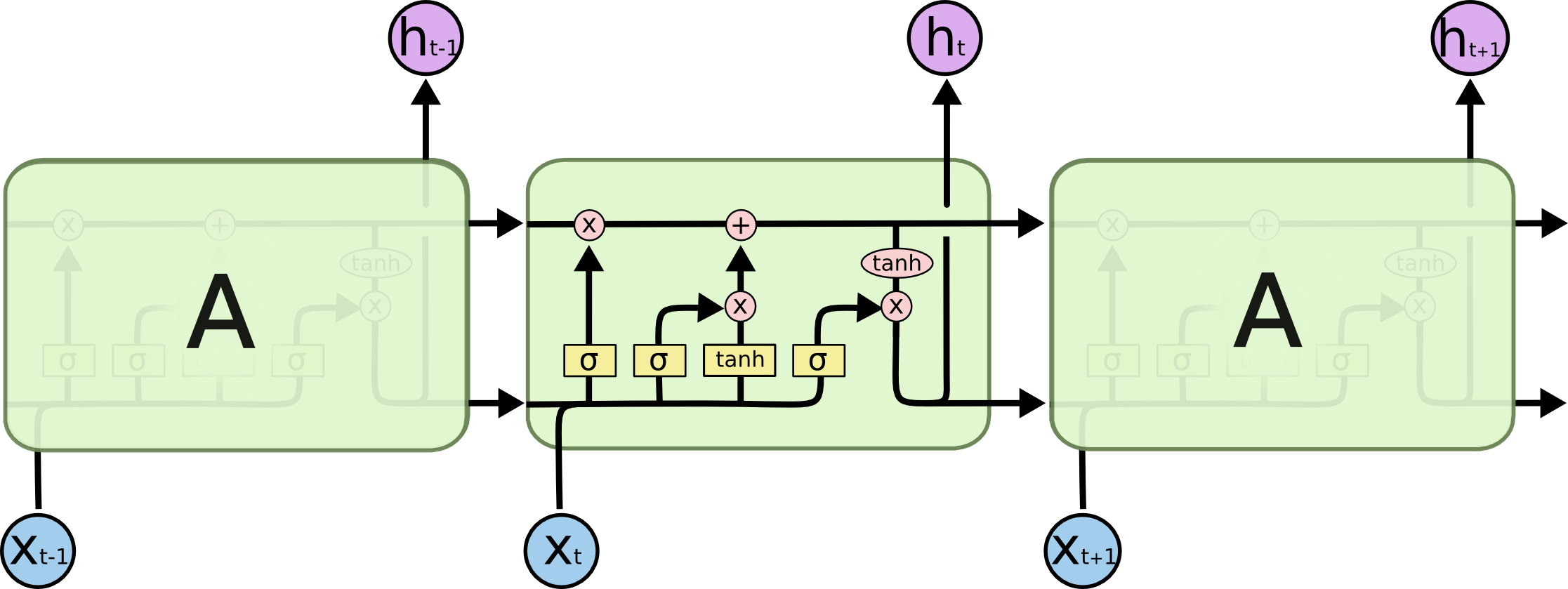# Model Geometry¶

This document explains several constants related to the geometry of an STT model.

## n_input¶

Each of the at maximum n_steps vectors is a vector of MFCC features of a time-slice of the speech sample. We will make the number of MFCC features dependent upon the sample rate of the data set. Generically, if the sample rate is 8kHz we use 13 features. If the sample rate is 16kHz we use 26 features… We capture the dimension of these vectors, equivalently the number of MFCC features, in the variable n_input. By default n_input is 26.

## n_context¶

As previously mentioned, the RNN is not simply fed the MFCC features of a given time-slice. It is fed, in addition, a context of $$C$$ frames on either side of the frame in question. The number of frames in this context is captured in the variable n_context. By default n_context is 9.

Next we will introduce constants that specify the geometry of some of the non-recurrent layers of the network. We do this by simply specifying the number of units in each of the layers.

## n_hidden_1, n_hidden_2, n_hidden_5¶

n_hidden_1 is the number of units in the first layer, n_hidden_2 the number of units in the second, and n_hidden_5 the number in the fifth. We haven’t forgotten about the third or sixth layer. We will define their unit count below.

The RNN consists of an LSTM RNN that works “forward in time”:The dimension of the cell state, the upper line connecting subsequent LSTM units, is independent of the input dimension.

## n_cell_dim¶

Hence, we are free to choose the dimension of this cell state independent of the input dimension. We capture the cell state dimension in the variable n_cell_dim.

## n_hidden_3¶

The number of units in the third layer, which feeds in to the LSTM, is determined by n_cell_dim as follows

n_hidden_3 = n_cell_dim


## n_hidden_6¶

The variable n_hidden_6 will hold the number of characters in the target language plus one, for the $$blank$$. For English it is the cardinality of the set

$\{a,b,c, . . . , z, space, apostrophe, blank\}$

we referred to earlier.